6. Algorithm of the Calculation in AsTher

Content
6.1. Behaviour of the System
6.1.1. Ideal gas - Clapeyron, 1834
6.1.2. Van der Waals, 1873
6.1.3. Redlich-Kwong, 1949
6.1.4. Generalized Redlich-Kwong, 1949
6.1.5. Redlich-Kwong Vapour-Liquid-Equilibrium, 1949
6.1.6. Soave Modification of Redlich-Kwong Vapour-Liquid-Equilibrium, 1972
6.1.7. Peng Robinson Vapour-Liquid-Equilibrium, 1976
6.2. State Functions
6.2.1. State functions in depend of specific heat capacity  and compressibility
6.2.2. Residual value of state function based on cubic equation of state
6.2.3. State functions based on correlation of measurements
6.3. Extrapolation
6.4. Calculation of the Equilibrium

6. 1. Behaviour of the System
When the critical constants Tc, Pc are known, the state functions can be calculated using real gases rules.
The behaviour of the system can be selected separately in each AsTher application.
The Generalized Redlich-Kwong equation of state (1949) is set as default.
Menu System -> Behaviour shows a window, in which the equation of state can be selected to calculation.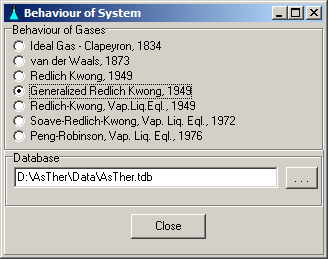6.1.1. Ideal gas - Clapeyron, 1834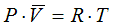State Functions are calculated corresponding to the equations in Cap. 6.2.1

6.1.2. Van der Waals, 1873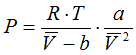a = 27 . R2 .Tc2/( 64 . Pc )
b =R . Tc /( 8 . Pc )

State Functions are calculated corresponding to the equations in Cap. 6.2.1

6.1.3. Redlich-Kwong, 1949a = 0.42748 . R2 .Tc5/2 / Pc

b = 0.08664 .R .Tc / Pc

State Functions are calculated corresponding to the equations in Cap. 6.2.1

6.1.4. Generalized  Redlich-Kwong, 1949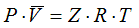a = 0.42748 . R2 .Tc2 / Pc
b = 0.08664 .R .Tc / Pc

h = 0.08664 .R .Tc /( Z . Pc
)

State Functions are calculated corresponding to the equations in Cap. 6.2.1

6.1.5. Redlich-Kwong Vapour-Liquid-Equilibrium, 1949

State Functions, compressibility and liquid volume are calculated corresponding to the equations in Cap. 6.2.2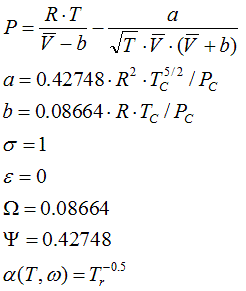6.1.6. Soave Modification of Redlich-Kwong Vapour-Liquid-Equilibrium, 1972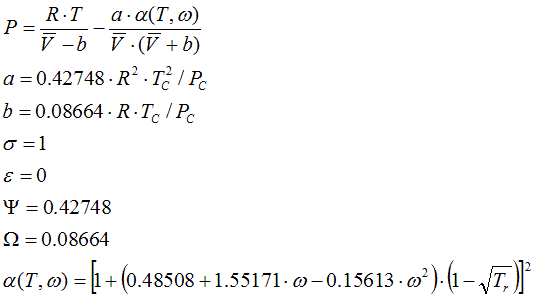α(T,ω) equation is due to Graboski and Daubert.

State Functions, compressibility and liquid volume are calculated corresponding to the equations in Cap. 6.2.2

6.1.7. Peng Robinson Vapour-Liquid-Equilibrium, 1976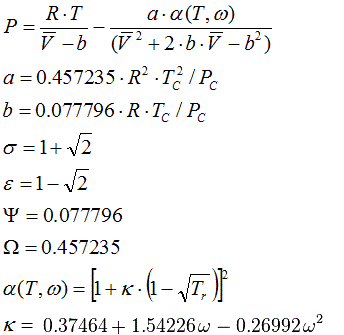State Functions, compressibility and liquid volume are calculated corresponding to the equations in Cap. 6.2.2

6.2. State Functions

Reference of the enthalpy values: enthalpy of elementar substances is zero at 25C and 1 bar in usual natural state.

The Cp �Function is calculated according to

Cp = a + 10-3 . b . T + 106 . c . T -2 + 10-6 . d . T2 + 109 . e . T -3 + 10-9 . f . T3
or
Cp =
a + b . (T/1000) + c . (T/1000) -2 + d . (T/1000)2 + e . (T/1000) -3f . (T/1000)3

a, b, c, d, e, f: empiric values in the AsTher Database from different sources, T [K]: Temperature.

If the constants are known according to the Shomate Equation
Cp = A + B .(T/1000) + C .(T/1000)� + D .(T/1000)� + E .(T/1000)-

then the constants can be replaced in AsTher database corresponding to the following table
Important: Ref.-Field in the dataset must not contain "[<hgt>]", when Shomate constants are used in a dataset

 Constants in Shomate Equation equal to the constants in AsTher database A a B b E c C d 0 (Zero) e D f

Important

The Constants for Shomate Equations from NIST can be used directly in Asther-Database,
only when the reference Enthalpy and Entropy are in the range of the validity of constants.

When the constants of the Cp function are valid at temperature 298 [K],
then the constants can be directly used in the AsTher Database.

When the constants of the Cp function are valid
at the temperature interval of 1000 -2000 [K],
then the constants can not be used in the AsTher Database

6.2.1. State functions in depend of specific heat capacity  and compressibility

The molar enthalpy is calculated according to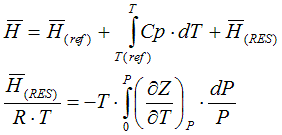The molar entropy is calculated according toThe molar free energy is  calculated according to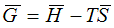6.2.2. Residual value of state function based on cubic equation of state
from Smith, Van Ness, and Abbott (Introduction to Chemical Engineering Thermodynamics, 7th ed.)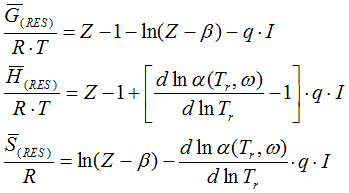Z: compressibility for vapours: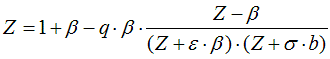Z: compressibility for liquids: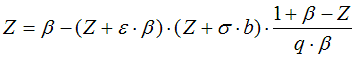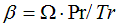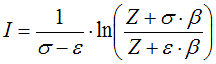6.2.3. State functions based on correlation of measurements

In several datasets, the calculations of H(T) and G(T) base on the measured data in small temperature range in non-ideal equilibrium state, without the Cp-correlation, when reference is [<hgt>].
In such case.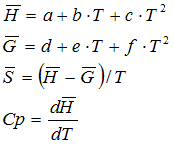a, b, c, d, e, f: empiric values in the AsTher Database according to different sources.

6.3. Extrapolation: when the extrapolation enabled, the calculations are carried out outsides of the data range.
When the extrapolation is not enabled, the calculations will be not are carried out and the reason is shown in the a messages window.

6.4. Calculation of the Equilibrium in the Application Equilibrium

The state of equilibrium is defined by following relations: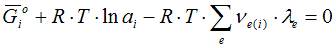or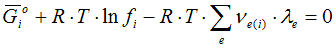Free enthalpy of the pure substance at temperature and pressure of the thermodynamic system
ai: Activity of the compound in the thermodynamic system
fi : Fugacity of the compound i in the thermodynamic system
R: Gas-constant T: Temperature
νe(i): Number of atoms of the element e in the compound i in the thermodynamic system
λe: Lagrange-Coefficient of the element e in the thermodynamic system

For the mass balance, following relations is valid:Ne: Mol number of the element e in the thermodynamic system
ni: Mol number of the compound i in the thermodynamic system
νe(i): Number of atoms of the element e in the compound i

All substances in the system will be treated within a mixed phase, if the activity coefficient of the substance is not set to (1) (in the a.c.-column). Consistency of a calculation is reached, when two criteria are fulfilled: - The mass balance can be closed. -
For any reaction the criteria of the equilibrium have to be fulfilled.
Evidence of the Correctness and Consistency:
Take any possible reaction within a given system:

a A (s) + b B (g) = c C (l)

First calculate the equilibrium constant K using the calculated values of the partial pressures and/or activities: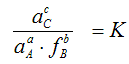then calculate the equilibrium constant K via the Gibbs (free) energy: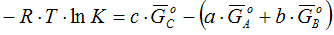Compare the K values from both equation
The Gibbs free energies can be calculated by the AsTher-Application Pure Substance at the given temperature of the system.
The change of the free energy can be calculated also by the AsTher-Application Reaction.
The results are allways within the selected accuracy.
Abs. 3.3. shows an example for the argumentation of consistency.